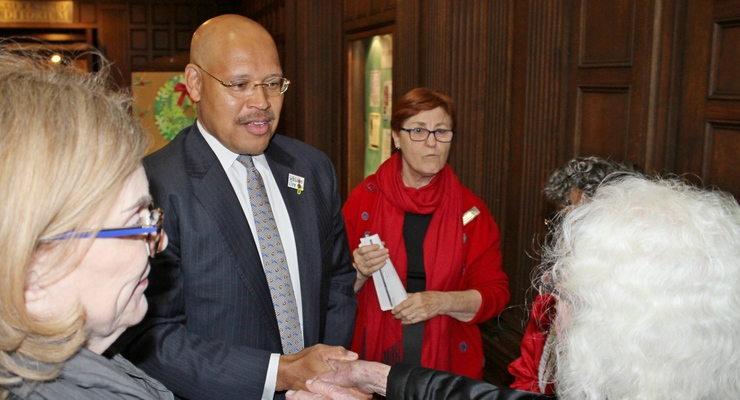New Caltech Professor Seeks Order in Chaos

A Q&A with Caltech's new mathematics professor Maksym Radziwill

Published : Tuesday, March 12, 2019 | 4:47 AMMaksym Radziwill, a new professor of mathematics at Caltech, has been fascinated by the question of randomness versus determinism since he was a teenager. “It’s phenomenal that you have things which somehow look very chaotic but are in fact predictable,” he says, explaining that math can be used to show that seemingly chaotic phenomena have order.

Radziwill studies number theory, a field that aims to understand properties of integers. He is particularly interested in the interactions of analytic number theory with other fields of mathematics, specifically probability, spectral theory, and harmonic analysis.

Born in Moscow and raised in Poland, Radziwill earned his bachelor’s degree in mathematics from McGill University in 2009 and his PhD from Stanford in 2013. He served as an assistant professor at Rutgers University and at McGill before coming to Caltech in 2018. He recently won the Breakthrough New Horizons in Mathematics Prize.

We sat down with Radziwill to talk about the meaning of math and how it applies to chaotic systems.

What made you decide to study number theory?
What drove me to number theory was its interactions with probability. Let’s take the distribution of primes, for example. Individually, it’s hard to predict if a number is a prime. For example, if we look at the number 1,027, it’s not clear immediately if it’s a prime. The same is true for 1,029—we don’t know if that’s a prime either [both numbers are in fact primes]. But if you look at the global distribution of primes or how often primes come up with subsequent numbers, that’s actually predictable. When something is complicated, it often looks random, but that does not mean it is.

Are you saying that randomness does not exist?
I am saying that what we perceive as randomness often emerges from having very complicated but deterministic rules. Here’s another example: You can generate “random numbers” on a computer, but since computers are deterministic, the numbers simply cannot be random even though they appear to be. It is very hard to tell for an external observer that the numbers come from predictable rules, but they do!

Can you give an example of how this applies to your research?
My colleague Kaisa Matomäki from the University of Turku, Finland, and I have done work on the factorization of integers into prime numbers. To factorize means to determine all of the prime numbers that divide a given number. What we have shown, roughly speaking, is that if you pick at random a big interval of numbers, say 10 to the power of 18 numbers, and then compare it to a small interval like 1,000 numbers and do the factorization of each set, then what you’ll very likely find is that the way integers factorize in this small interval is similar to the way they factorize in the big interval. There are no hidden biases. So factorization, a purely deterministic process, behaves in a fairly random way.

Does this finding have any real-world applications?
This result is reassuring for our cryptographic algorithms, which rely on the assumption that factorization is so complex that it is essentially random. For example, the idea behind the “RSA” public key cryptography protocol is to pick two prime numbers, which are your secret key. When you multiply them, this becomes the public key, which can be shared in the open. Because factorizing this number back into primes is very difficult, one cannot easily recover your private key from the public key. Somebody who has your public key can encrypt a message, but only the owner of the private key can decrypt the message. If there were some hidden biases in the factorization of integers, then that could give a potential attacker a small edge in recovering these two primes that constitute the secret key, thus allowing them to decrypt the message.

•••